• 这里我利用的是matplotlib.pyplot.plot的工具来绘制折线，这里先给出个段代码和结果： # -*- coding: UTF-8 -*- import numpy as np import matplotlib as mpl import matplotlib.pyplot as plt #这里导入...
这里我利用的是matplotlib.pyplot.plot的工具来绘制折线图，这里先给出一个段代码和结果图：

# -*- coding: UTF-8 -*-
import numpy as np
import matplotlib as mpl
import matplotlib.pyplot as plt

#这里导入你自己的数据
#......
#......
#x_axix，train_pn_dis这些都是长度相同的list()

#开始画图
sub_axix = filter(lambda x:x%200 == 0, x_axix)
plt.title('Result Analysis')
plt.plot(x_axix, train_acys, color='green', label='training accuracy')
plt.plot(sub_axix, test_acys, color='red', label='testing accuracy')
plt.plot(x_axix, train_pn_dis,  color='skyblue', label='PN distance')
plt.plot(x_axix, thresholds, color='blue', label='threshold')
plt.legend() # 显示图例

plt.xlabel('iteration times')
plt.ylabel('rate')
plt.show()
#python 一个折线图绘制多个曲线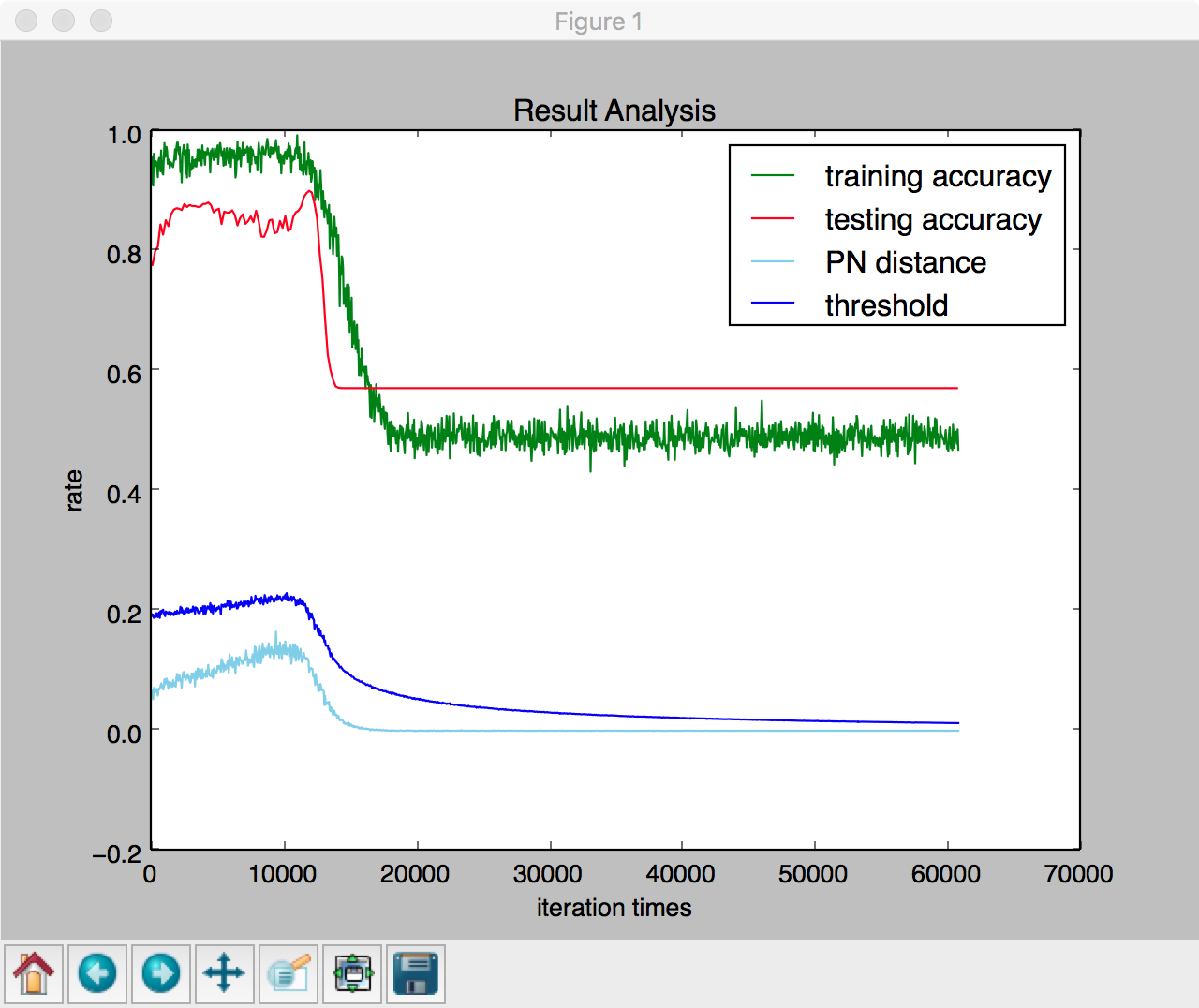这里我谈谈matplotlib.pyplot.plot()的使用方法，先附上一个官方文档链接，然后我说下可能用到的一些参数，参数可选的内容我就不一一展开了，大家可以去上面那个连接里查：

color：曲线颜色，blue，green，red等等
label：图例，这个参数内容就自定义啦，注意如果写这个参数一定要加上plt.legend()，之后再plt.show()才有有用！
linestyle：曲线风格，’–’，’-.’，’:’等等
linewidth：曲线宽度，自定义就可以
marker：标记点样式，’o’,’x’，也就是说这些符号会标示出曲线上具体的“点”，这样一来就易于观察曲线上那些地方是支撑点
markersize：标记点的大小，自定义就可以
如果不希望画到同一张图, 可以加一句:

plt.cla()

plt.legend()如果要显示label必须加
展开全文• python使用matplotlib绘图详解（自动标记、将线绘制在中，绘制张图） plt.plot(x,y,markes,label)，label表示图例，x为横坐标，y为纵坐标，markes为标记 使用for循环将条线段绘制在中 ...
python使用matplotlib绘图详解（自动标记、将多条线绘制在一个图中，绘制多张图）
plt.plot(x,y,markes,label)，label表示图例，x为横坐标，y为纵坐标，markes为标记

使用for循环将多条线段绘制在一个图中
import numpy as np
import matplotlib.pyplot as plt
num = np.arange(40).reshape(10,4)#生成10行4列的数组
plt.rcParams['font.sans-serif'] = ['SimHei']#可以plt绘图过程中中文无法显示的问题
plt_label = 0
for link in range(len(num)):
plt_label += 1
plt.plot(num,num[link],label = '第'+ str(plt_label) + '条线段')
plt.legend()#显示图例，如果注释改行，即使设置了图例仍然不显示
plt.show()#显示图片，如果注释改行，即使设置了图片仍然不显示



结果：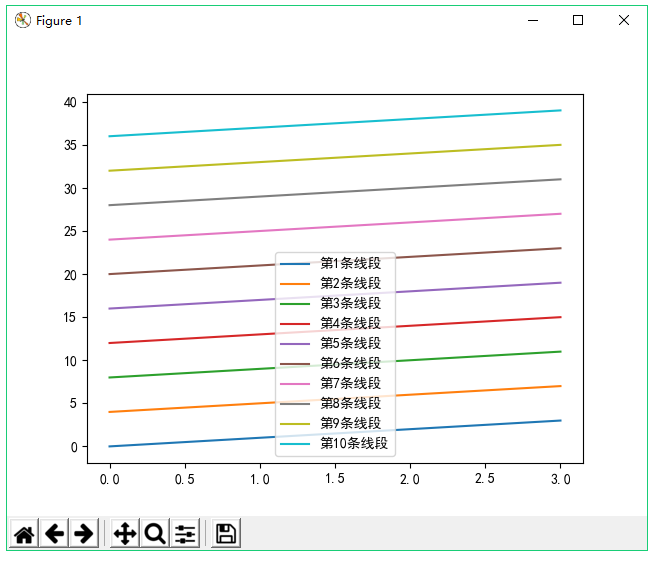为了更好的区分直线，可能需要对数据点进行标记，标记可以使用markes

import numpy as np
import matplotlib.pyplot as plt
num = np.arange(40).reshape(10,4)#生成10行4列的数组
plt.rcParams['font.sans-serif'] = ['SimHei']#可以解释中文无法显示的问题
plt_label = 0
for link in range(len(num)):
plt_label += 1
markes = ['-o', '-s', '-^', '-p', '-^', '-v', '-p', '-d', '-h', '-2', '-8', '-6']
plt.plot(num,num[link],markes[link],label = '第'+ str(plt_label) + '条线段')
plt.legend()
plt.show()


结果：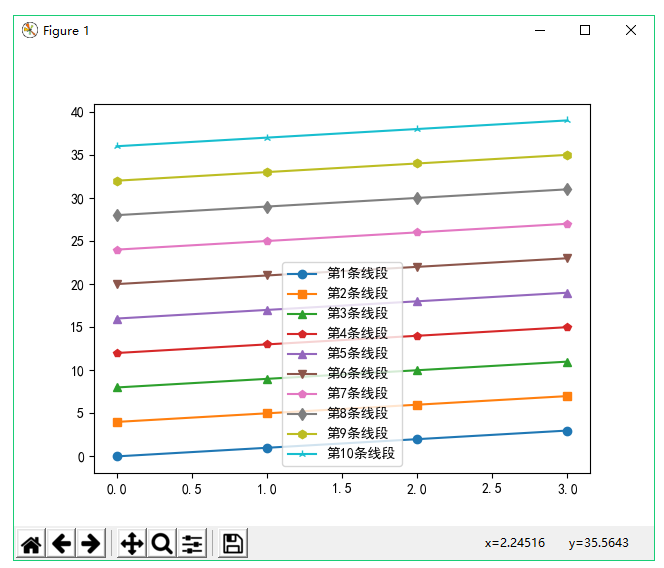另外如果你感觉一张图中绘制的直线过多，想要每个图中绘制五条直线，那么你可以使用figure（）：
import numpy as np
import matplotlib.pyplot as plt
num = np.arange(40).reshape(10,4)#生成10行4列的数组
plt.rcParams['font.sans-serif'] = ['SimHei']#可以解释中文无法显示的问题
plt_label = 0
for link in range(len(num)):
plt_label += 1
markes = ['-o', '-s', '-^', '-p', '-^', '-v', '-p', '-d', '-h', '-2', '-8', '-6']
half = len(num)/2
if link < half:
plt.figure('第一个图片')
plt.plot(num,num[link],markes[link],label = '第'+ str(plt_label) + '条线段')
plt.legend()
else:
plt.figure('第二个图片')
plt.plot(num,num[link],markes[link],label = '第'+ str(plt_label) + '条线段')
plt.legend()
plt.show()


结果：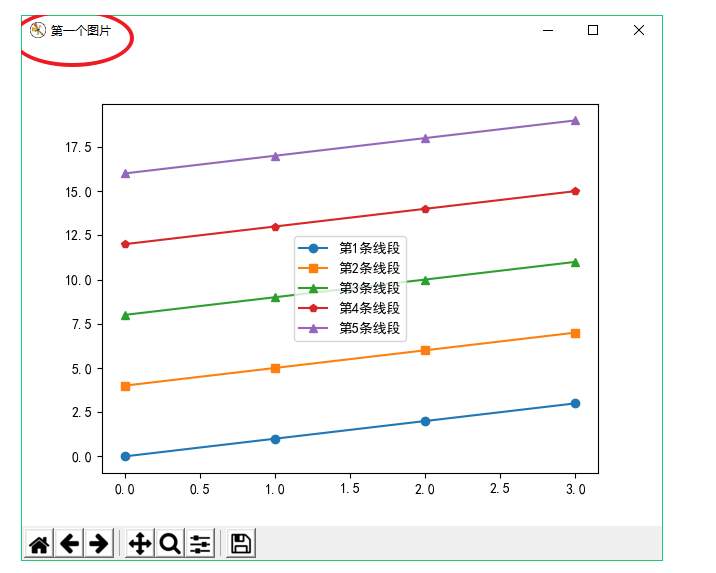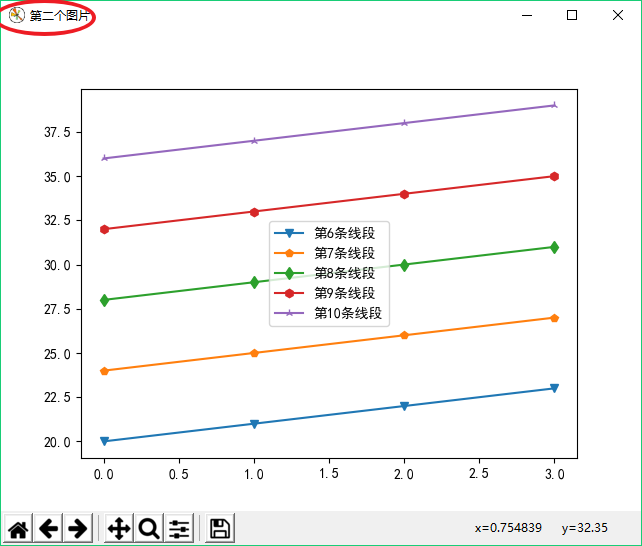展开全文python绘图
• matplotlib 中，个figure即为个画板，用plt.figure()创建个新画板，如果只有个画板的话这句可以省略。 #创建第个视图（画板） plt.figure(1) #第个画板的内容 #… #创建第二个视图（画板） plt.figure...
1、生成多个视图（画板）：
在matplotlib 中，一个figure即为一个画板，用plt.figure()创建一个新画板，如果只有一个画板的话这句可以省略。
#创建第一个视图（画板）
plt.figure(1)
#第一个画板的内容
#…
#创建第二个视图（画板）
plt.figure(2)
#第二个画板的内容
#…
#切换到第一个画板
plt.figure(1)
#继续第一个画板的内容
#…
2.一个视图中绘制多个子图：
在matplotlib 中，一个figure视图可以包括多个子图（Axes），通过subplot() 函数实现。

subplot(numRows, numCols, plotNum)

numRows声明视图中子图有多少行，numCols声明视图中子图有多少列，plotNum则声明此时进行绘制的是第一个子图。子图的序号按照以行优先的原则，从左到右，从上到下。
如：subplot(2,2,3)表示开始绘制4个子图的第3个（左下角位置）子图（蓝色）。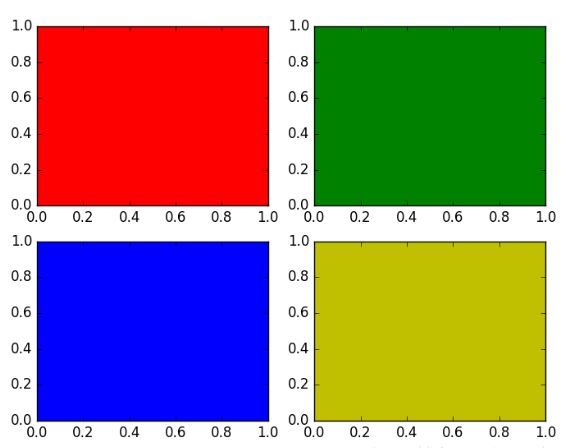3.绘制多条曲线：
import matplotlib.pyplot as plt
from matplotlib.collections import EventCollection
import numpy as np
# Fixing random state for reproducibility
np.random.seed(19680801)
#定义好两条曲线的数据
xdata = np.random.random([2, 10])
xdata1 = xdata[0, :]
xdata2 = xdata[1, :]
xdata1.sort()
xdata2.sort()
ydata1 = xdata1 ** 2
ydata2 = 1 - xdata2 ** 3
#绘制图画
fig = plt.figure()                         #生成一个画板
ax = fig.add_subplot(1, 1, 1)              #定义有着1行1列子图的视图并取第一个
ax.plot(xdata1, ydata1, color='tab:blue')  #第一条曲线
ax.plot(xdata2, ydata2, color='tab:orange')#第二条曲线

# 定义坐标轴范围
ax.set_xlim([0, 1])
ax.set_ylim([0, 1])

ax.set_title('line plot with data points')
plt.show()

结果如下：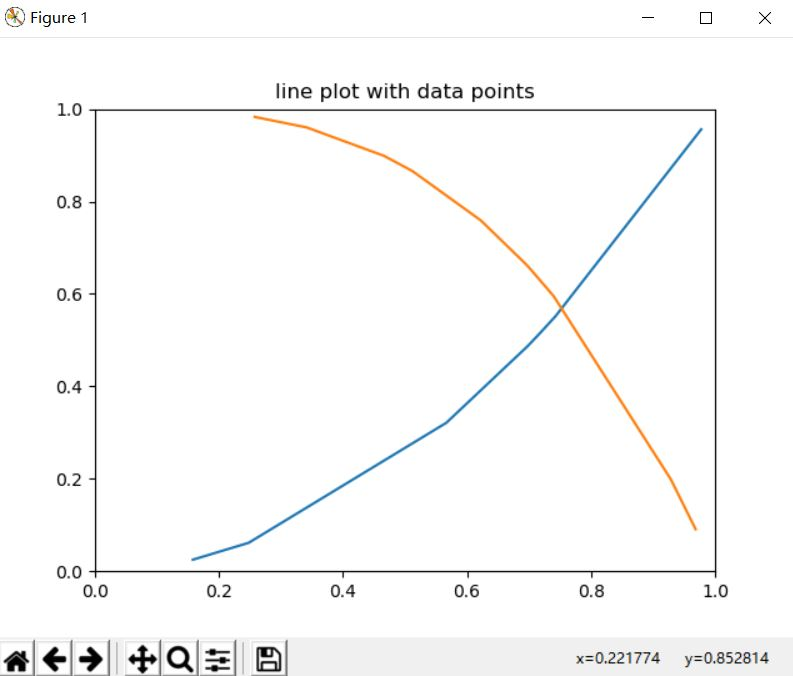4.下面给一个综合的例子：
import numpy as np
import matplotlib.pyplot as plt

#定义好横纵坐标数据
x1 = np.linspace(0.0, 5.0)
x2 = np.linspace(0.0, 2.0)
y1 = np.cos(2 * np.pi * x1) * np.exp(-x1)
y2 = np.cos(2 * np.pi * x2)

plt.figure(1)                           #第一个画板
ax1=plt.subplot(2, 1, 1)                #构建2行1列的视图，选定第一个子图
ax1.plot(x1, y1, color='tab:blue')      #第1条曲线
ax1.plot(x2, y2, color='tab:orange')    #第2条曲线
ax1.set_title('ax1 with two lines')     #子图1的标题

ax2=plt.subplot(2, 1, 2)                #在2行1列的视图中，选定第二个子图
ax2.plot(x1, y1, color='tab:blue')
ax2.set_title('ax2 with one line')      #子图2的标题
plt.xlabel('x label')
plt.ylabel('y label')
plt.suptitle('A tale of 2 subplots')    #该视图的标题

plt.figure(2)                           #第2个画板
ax2=plt.subplot(2, 1, 2)
ax2.plot(x1, y1, color='tab:blue')
plt.show()

结果如图：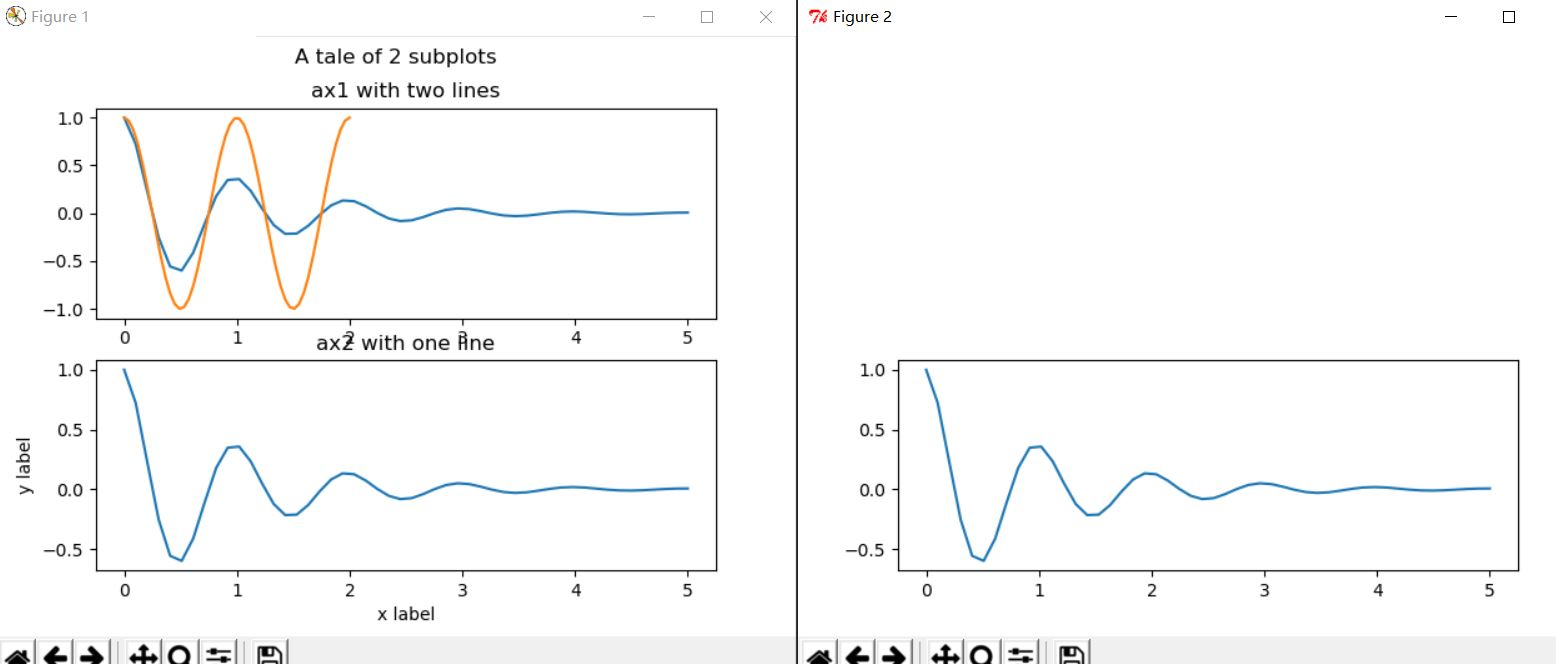5.子图的不规则划分：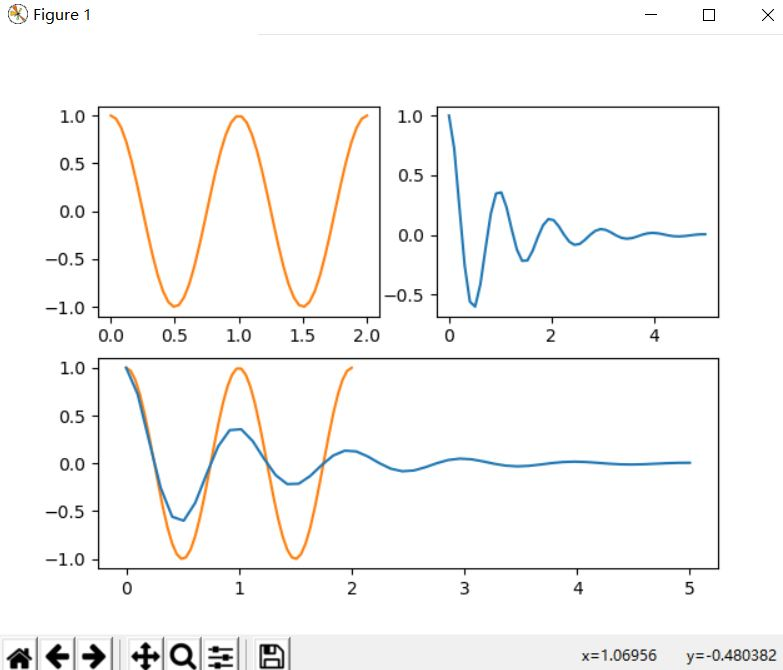将整个图按照2 * 2划分，前两个为plt.subplot(2,2,1)和plt.subplot(2,2,2),最后一个需要对整个图进行重新划分，按照2 * 1划分，为plt.subplot(2,1,2)
import numpy as np
import matplotlib.pyplot as plt

#定义好横纵坐标数据
x1 = np.linspace(0.0, 5.0)
x2 = np.linspace(0.0, 2.0)
y1 = np.cos(2 * np.pi * x1) * np.exp(-x1)
y2 = np.cos(2 * np.pi * x2)

ax1=plt.subplot(2, 2, 1)
ax1.plot(x2, y2, color='tab:orange')

ax2=plt.subplot(2, 2, 2)
ax2.plot(x1, y1, color='tab:blue')

ax3=plt.subplot(2, 1, 2)
ax3.plot(x2, y2, color='tab:orange')
ax3.plot(x1, y1, color='tab:blue')
plt.show()



展开全文python 数据可视化 可视化
• matplotlib 的 figure 就是个 单独的 figure 小窗口, 小窗口里面还可以有更的小图片. 使用import导入模块matplotlib.pyplot，并简写成plt 使用import导入模块numpy，并简写成np import matplotlib.pyplot as plt...
转载请注明：虚幻私塾 » matplotlib figure 图像
简单的线条
matplotlib 的 figure 就是一个 单独的 figure 小窗口, 小窗口里面还可以有更多的小图片.
使用import导入模块matplotlib.pyplot，并简写成plt 使用import导入模块numpy，并简写成np
import matplotlib.pyplot as plt
import numpy as np

使用np.linspace定义x：范围是(-3,3);个数是50. 仿真一维数据组(x ,y1)表示曲线1. 仿真一维数据组(x ,y2)表示曲线2.
x = np.linspace(-3, 3, 50)
y1 = 2*x + 1
y2 = x**2

使用plt.figure定义一个图像窗口. 使用plt.plot画(x ,y1)曲线.
plt.figure()
plt.plot(x, y1)
plt.show()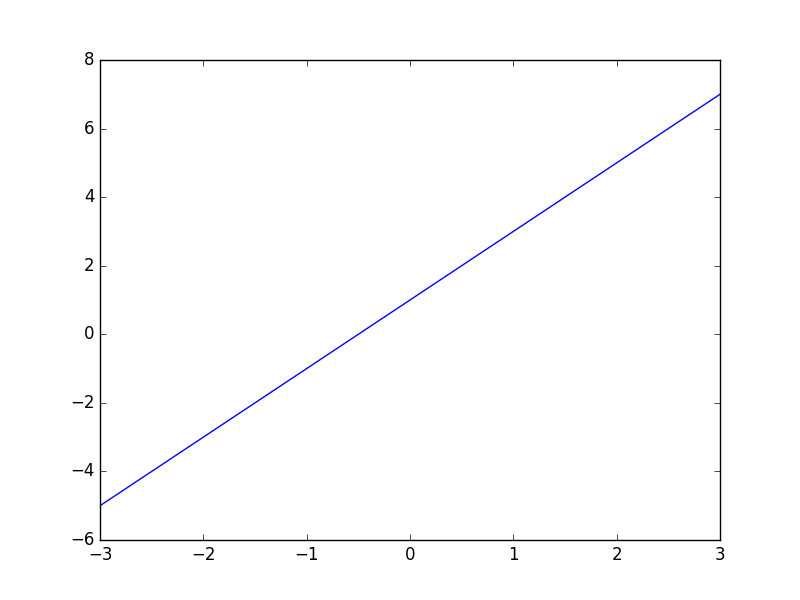使用plt.figure定义一个图像窗口：编号为3；大小为(8, 5). 使用plt.plot画(x ,y2)曲线. 使用plt.plot画(x ,y1)曲线，曲线的颜色属性(color)为红色;曲线的宽度(linewidth)为1.0；曲线的类型(linestyle)为虚线. 使用plt.show显示图像.
plt.figure(num=3, figsize=(8, 5),)
plt.plot(x, y2)
plt.plot(x, y1, color='red', linewidth=1.0, linestyle='--')
plt.show()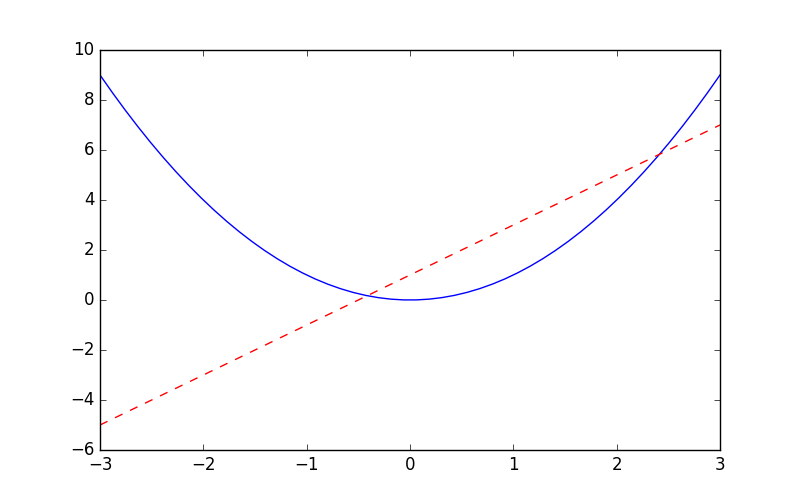展开全文• 目录：1、准备工作2、示例演示3、添加标签4、改变线条的风格5、绘制线条6、改变整体的风格1、准备工作首先要确保安装好Matplotlib库用pip下载，打开cmd输入pip install matplotlib即可完成下载2、示例演示展示的...
• 就是连个y值个x值 import matplotlib.pyplot as plt from matplotlib import font_manager import random x= range(1,27,2) y1 = [random.randint(1,30) for i in x] y2 = [random.randint(1,30) for i in...
• 要在同里面绘制线，只需要创建个figure，然后不断的进行plot绘制线图，以及线对应的参数设置（可选操作），最后show()一下就可以了： import matplotlib.pyplot as plt import random x = list(range(10...python 数据可视化 数据分析
• 做数据分析，还有机器学习的收敛性，准确性分析时，往往需要将一些数据图形化，以曲线的形式显示出来，下面就介绍两种方式来实现这个小问题，种是object-oriented面向对象的，另种是基于plt的。 下面这个方式是...
• 今天想直观的展示一下数据就用到了matplotlib模块，之前都是一张只有条曲线，现在想同个图片上绘制条曲线来对比，实现很简单，具体如下：#!usr/bin/env python #encoding:utf-8 ''' __Author__:沂水寒城 ...
• plt.subplots()的作用是创建或者组子图返回值是Figure，axes.Axes。此处代码中 fig = Figure ax = axes.Axes。 3.使用Axes.plot作图 fig, ax = plt.subplots() # 创建实例 x = np.linspacpython 数据可视化 plot
• 今天想直观的展示一下数据就用到了matplotlib模块，之前都是一张只有条曲线，现在想同个图片上绘制条曲线来对比，实现很简单，具体如下： #!usr/bin/env python #encoding:utf-8 ''' __Author__:沂水寒城 ...
• matplotlib画图时，常常需要实现两类功能，类是在个坐标轴上画条曲线，能够清楚地看到条曲线的对比情况。另种情况是在同个窗口的不同子图上画图，用于呈现不同内容的曲线，没有对比关系的曲线。...Python 数据可视化
• 今天想直观的展示一下数据就用到了matplotlib模块，之前都是一张只有条曲线，现在想同个图片上绘制条曲线来对比，实现很简单，具体如下：#!usr/bin/env python#encoding:utf-8'''__Author__:沂水寒城功能：...
• 收下这份来自GitHub的神器，一图搞定Matplotlib！ 觉得有用的话,欢迎一起讨论相互学习~ 作　者：早起Python 出　处：https://www.cnblogs.com/liuzaoqi/p/12769593.html 今天的推送没有太废话，给大家分享一位...
• 先给出效果： 制作方法： 最近被被要求画出如下的若干段脑电信号在同一张图内并行陈列的图表 这幅的横坐标单位是...然而这幅个缺点，就是横坐标没办法显示成确切的时间点，如完善11点01分11秒这...Python 可视化
• 大家好，最近在研究在搞Python的大作业，有个...1、subplot合一其实，利用python 的matplotlib包下的subplot函数可以将个子图放在同个画板上。在此之前，我们先来看个案例：import matplotlib.pyplot as p...
• 公众号关注“ML_NLP”设为 “星标”，重磅干货，第时间送达！作者：禹棋赢本文推荐个仓库Plot-Pictures-Tutorial-...每个plot函数对应条曲线，画线的时候调用个plot函数即可。plot()函数：前两个参数...
• matplotlib 的 figure 就是个 单独的 figure 小窗口, 小窗口里面还可以有更的小图片. 使用import导入模块matplotlib.pyplot，并简写成plt使用import导入模块numpy，并简写成np import matplotlib.pyplot as plt ...
• 原博文2019-07-14 16:13 −在讲解绘制个子图之前先简单了解一下使用matplotlib绘制，导入绘图所需库matplotlib并创建个等间隔的列表x，将[0,2*pi]等分为50等份，绘制函数sin(x)。当没有给定x轴数值时，...
• 使用matplotlib绘制折线 　 直接使用plot()函数 　plt.plot(x,y,format_string,**kwargs) 　x轴数据，y轴数据，format_string控制曲线的格式字串，format_string由颜色字符，风格字符，和标记字符 。　 导入库 ...python 可视化 数据分析
• 掌握好初级的可视化，能够在数据分析的过程中利用可视化帮助...网络上已经有太优秀的matplotlib的教程了，不想花太时间出重复的教程。因此本文不是篇正经的教程，仅作为最近读完《Matplotlib for Python Dev...
•   这里我利用的是matplotlib.pyplot.plot的工具来绘制折线，这里先给出个段代码和结果： # -*- coding: UTF-8 -*- import numpy as np import matplotlib as mpl import matplotlib.pyplot as plt #这里...
• 今天想直观的展示一下数据就用到了matplotlib模块，之前都是一张只有条曲线，现在想同个图片上绘制条曲线来对比，实现很简单，具体如下：#!usr/bin/env python#encoding:utf-8'''__Author__:沂水寒城功能：......